Learnyst Support

Now you can add mathematical equations to your discussion replies. And it is as easy as a hot knife through butter. Here's how you can do it,

• Open the discussion from the manage tab.• Open the discussion forum, select the unanswered question and click on the reply button.• Go to the website: https://arachnoid.com/latex/ and this takes you to an interactive latex builder that looks like this.• Refer the how to add math equations to your online quiz support article to create your math equation.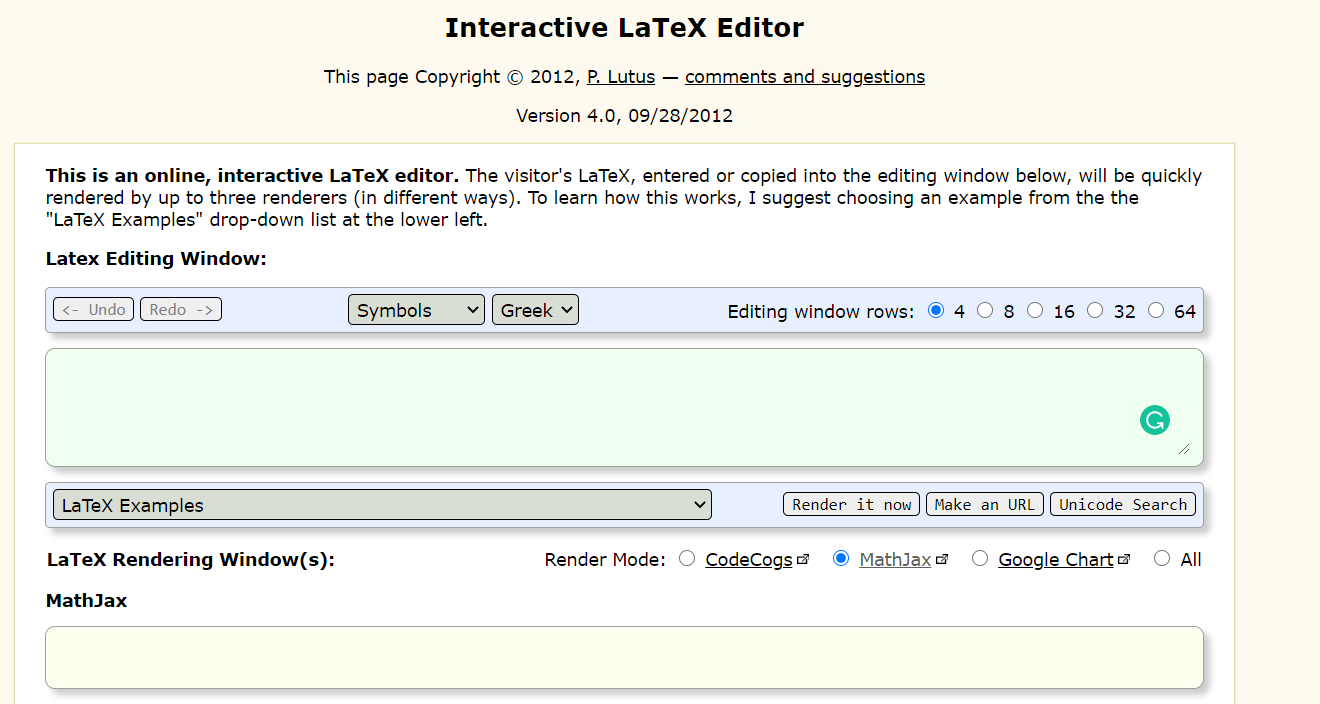• Now, use either the inline syntax as shown in the image to paste your equations in the discussion forum.• This is how the inline form of the equation will appear: $$3x^2-4\sqrt{3}+4=0$$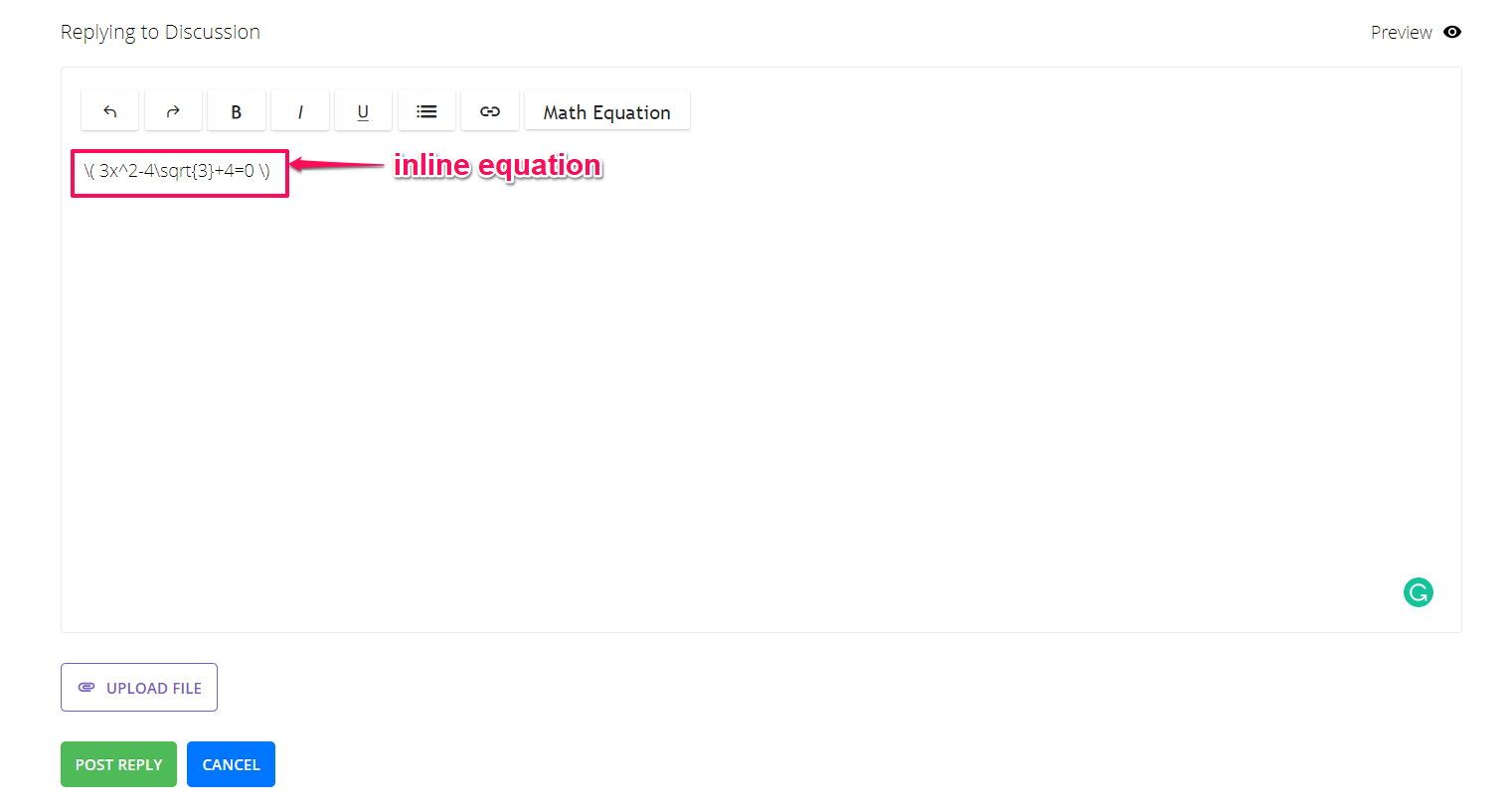• Alternatively, you can use the square brackets to display your equation on a separate line $3x^2-4\sqrt{3}+4=0$

• Once you have added the equation, click on the post reply button to publish your math equation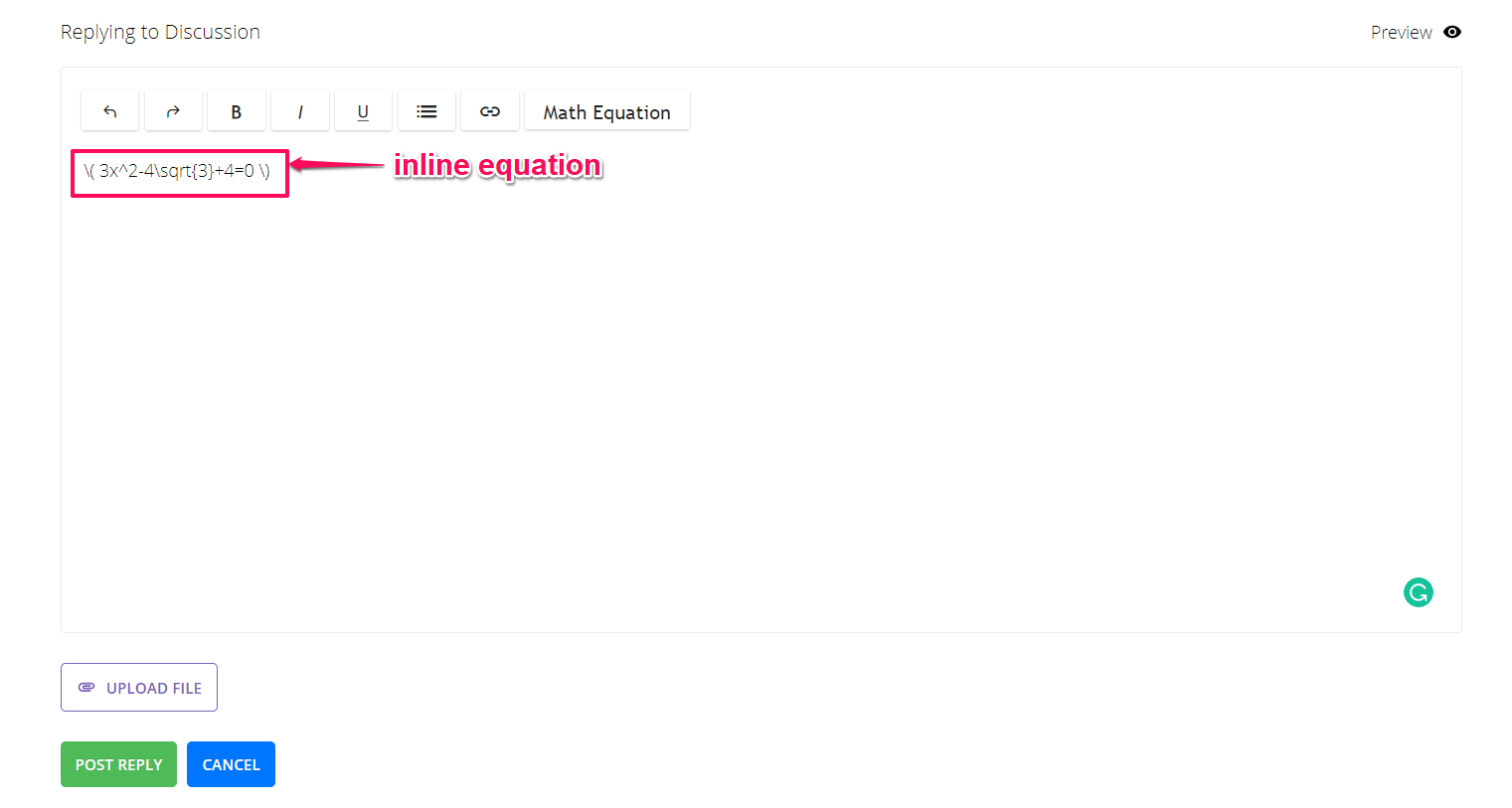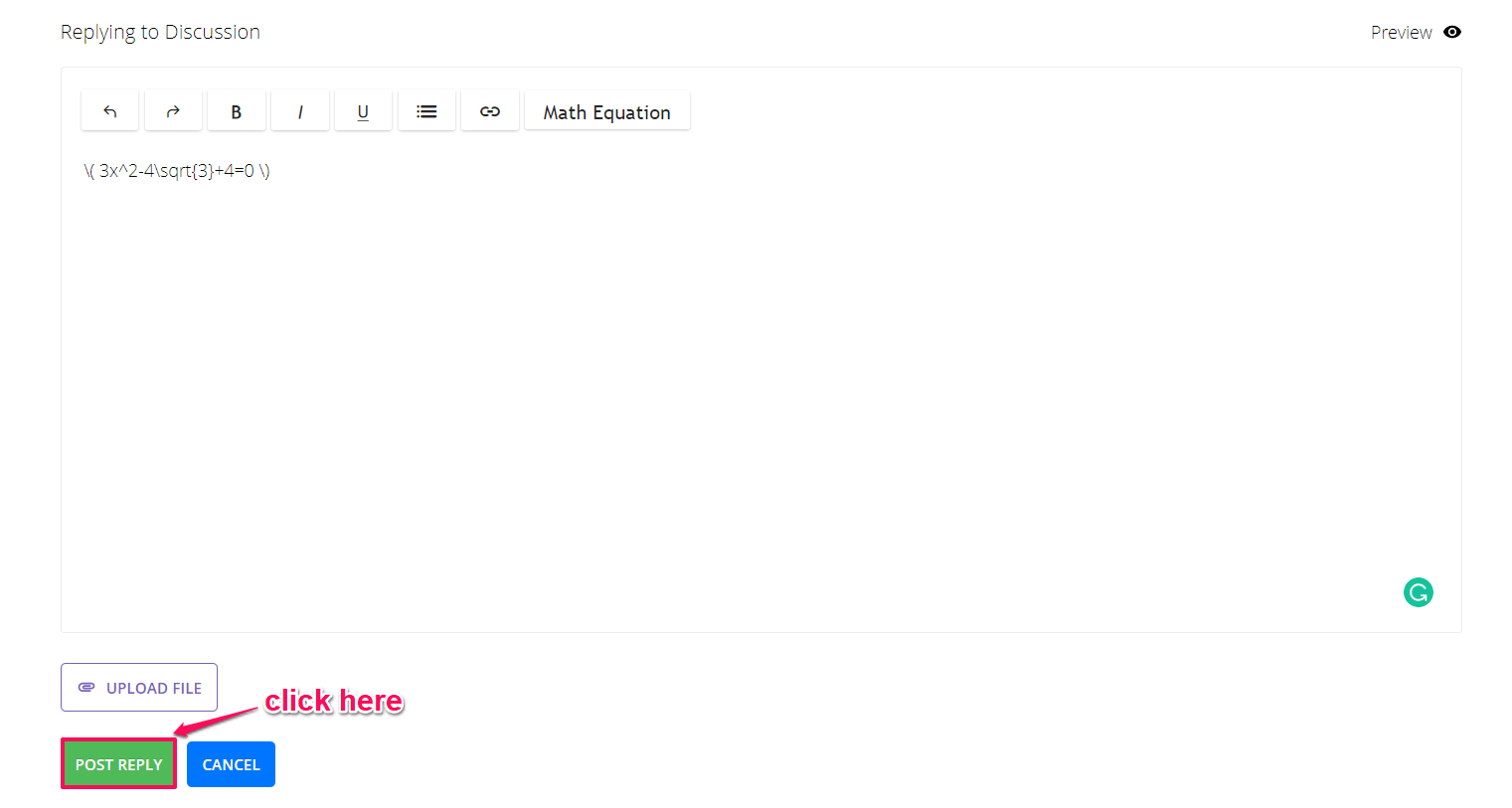This is how the final equation will appear to your student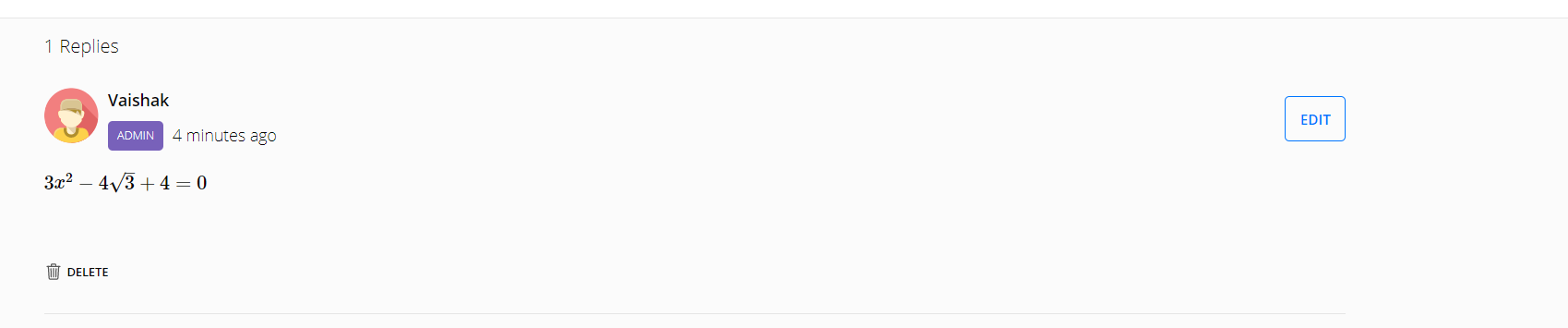Hope this support article, helped you to add math equations in your discussion forums.

Do check out the complete guide to adding math equations to your online quiz to know more details.